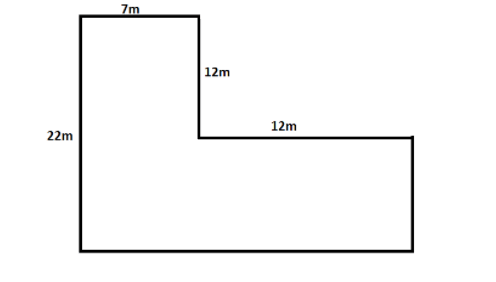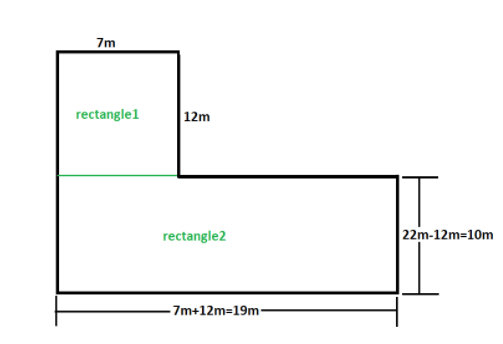Courses
Courses for Kids
Free study material
Offline Centres
MoreLast updated date: 23rd Nov 2023
Total views: 279.9k
Views today: 3.79k

# Find the area of the given figure:Verified
279.9k+ views
Hint: Here though the figure appears to be not that good it has two hidden rectangles if we observe it carefully. And finding the area of those two rectangles will give the area of the full figure. We will redraw the figure according to our convenience and then use the formula of area of rectangles. Adding those areas will give the total area.

We will redraw the figure as the figure given below and also label the dimensions.Now we will find the area of the rectangles.
For rectangle1:
Length = 7m
Thus area is given by;
$\operatorname{Re} ct1 = l \times b = 7 \times 12 = 84{m^2}$
For rectangle2:
Length = 19m
$\operatorname{Re} ct2 = l \times b = 19 \times 10 = 190{m^2}$
$= 84 + 190 = 274\;{m^2}$
Thus the total area is $274\;{m^2}$.
So, the correct answer is “$274\;{m^2}$”.